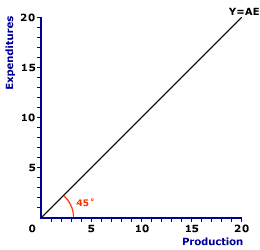Saturday  June 25, 2022
 AmosWEB means Economics with a Touch of Whimsy!AGGREGATE EXPENDITURE DETERMINANT: A ceteris paribus factor that affects aggregate expenditures, but which is assumed constant when the aggregate expenditure line is constructed. Changes in any of the aggregate expenditures determinants cause the aggregate expenditure line to shift. While a wide variety of specific ceteris paribus factors can cause the aggregate expenditure line to shift, it's usually most convenient to group them into the four, broad expenditure categories -- consumption, investment, government purchases, and net exports. The reason is that changes in these expenditures are the direct cause of shifts in the aggregate expenditure line. If any determinant affects aggregate expenditures it MUST affect one of these four expenditures.45-DEGREE LINE:

A line that shows equality between the variable measured on the vertical axis of a diagram and the variable measured on the horizontal axis. A 45-degree line is used both as a guideline that provides insight into the measured variables and as a critical part of the analysis. This line is perhaps most important in Keynesian economics. It is used in a diagram measuring consumption and income as a means of deriving saving---the difference between income and consumption. It is also used in the Keynesian model (or Keynesian cross) diagram measuring aggregate expenditures and aggregate production as a means of identifying equilibrium--the equality between aggregate expenditures and aggregate production.
45-Degree LineThe 45-degree line assists in the Keynesian economics evaluation and analysis of the macroeconomy. In general, a 45-degree line is so named because it forms a 45-degree angle with both the vertical or horizontal axes of a typical right-angle diagram. This is achieve because each point on the 45-degree line equates the variable measured on the vertical axis with the variable measured on the horizontal axis. Because the two variables are measured in the same units, the 45-degree line has a slope of one and it bisects the 90-degree angle formed by the vertical and horizontal axes.

A typical 45-degree line is presented in the exhibit to the right. In this particular exhibit, expenditures (aggregate expenditures) are measured on the vertical axis and production (aggregate production) is measured on the horizontal axis. The 45-degree line then depicts each point in this diagram in which aggregate production (Y) is equal to aggregate expenditures (AE). For this reason, the 45-degree line is also labeled Y=AE.

The two most common uses of the 45-degree line are the Keynesian model and the consumption line.

• Keynesian Model: The most important use of the 45-degree is the Keynesian model, which identifies equilibrium equality between aggregate expenditures and aggregate production. In particular, the intersection between the 45-degree line and the aggregate expenditures line indicates the equilibrium level of aggregate production.

• Consumption Line: The 45-degree line is also used as a guide for working with the consumption line. Because the 45-degree line has a slope of one, it serves to highlight that the slope of the consumption line is less that one. Moreover, because the 45-degree in this diagram shows the equality between consumption and income, it can be used to easily identify saving as the vertical difference between the consumption line an the 45-degree line.Recommended Citation:

45-DEGREE LINE, AmosWEB Encyclonomic WEB*pedia, http://www.AmosWEB.com, AmosWEB LLC, 2000-2022. [Accessed: June 25, 2022].

Check Out These Related Terms...

Or For A Little Background...

And For Further Study...
Search Again?PURPLE SMARPHIN[What's This?] Today, you are likely to spend a great deal of time searching for a specialty store hoping to buy either a video game player or an AC adapter that won't fry your computer. Be on the lookout for telephone calls from former employers.Your Complete ScopeThe New York Stock Exchange was established by a group of investors in New York City in 1817 under a buttonwood tree at the end of a little road named Wall Street."It is not fair to ask of others what you are unwilling to do yourself. "-- Eleanor Roosevelt, diplomat, activistBLSBureau of Labor StatisticsA PEDestrian's Guide Xtra CreditTell us what you think about AmosWEB. Like what you see? Have suggestions for improvements? Let us know. Click the User Feedback link.| | | | | | | | | | |
| | | |

Thanks for visiting AmosWEB